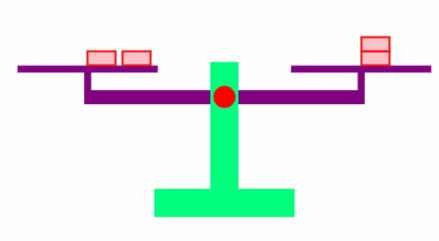AM
Animated Mathematics

### Svenska# Algebra is Based on Balance

An algebraic equation has an equal sign, where the left hand side (LHS) equals the right hand side (RHS):

Equation where the left hand side equals the right hand side. Required for all equations.

This is the same balance seen in a traditional weighing machine also known as a set of scales. A set of virtual scales is shown below:

Interactive animation of a balance scale to explain the fundamentals of algebra

You can use the buttons to add and subtract bricks on the left and right scales. The balance is level when the weight on the left and right are equal. Balance is maintained so long as you add/subtract the same amount on the left and right; this is the basis of algebra. Lines of advanced algebra can look complicated, however one can have confidence that its can be understood, since every step is based on a very simple and easy to visualise concept, namely the balance scale.

### Exercise: One Step Problem

The gold bricks weigh 1g each; by removing gold bricks you determine the weight of the pink brick. The buttons allows you to add or subtract gold bricks from both sides (to ensure balance).

In an equation, the unknown (which in this case is the pink brick) is defined as "x". Formally, finding the value of x is known as "solving the equation". The above balance problem is equivalent to a "one step equation"

### Exercise: Two Steps Problem

The gold bricks weigh 1g each; by removing gold and pink bricks you determine the weight of a single pink brick. The buttons allows you to add or subtract gold/pink bricks from both sides.

Gold:

Pink:

The weight of the pink brick is the unknown labelled "x". The above balance problem is equivalent to a "two step equation":

### Exercise: Solve Two Steps Problem

Solve the following two step problem on paper and show your working out correctly.

x value

You may get a negative value for x and you are recommended to substitute your answer into the original equation to check your answer. You can check your working out below:

#### Summary

The rules of Algebra are based on a balance scale. An equation is formed by naming the unknown quantity on the balance scale as "x", and then an equation which has a left hand side (LHS), a right hand side (RHS), and an equal sign is formed by looking at the items on the balance scale. We can add/subtract a quantity from the LHS so long as we add/subtract the same quantity from the RHS; this can be repeated many times until we find the value of x. The x value can then substituted back into the original formula, and if you find that LHS=RHS, then you can be confident that your algebraic steps are correct.